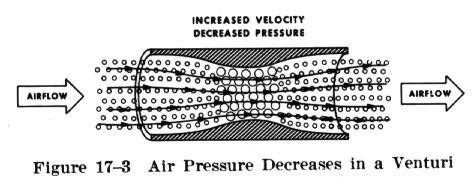Bernoulli's Principle of Pressure Bernoulli's Principle of Pressure

A half century after Sir Newton presented his laws, Mr. Daniel Bernoulli, a Swiss mathematician, explained how the pressure of a moving fluid (liquid or gas) varies with its speed of motion. Specifically, he stated that an increase in the speed of movement or flow would cause a decrease in the fluid's pressure. This is exactly what happens to air passing over the curved top of the airplane wing.

An appropriate analogy can be made with water flowing through a garden hose. Water moving through a hose of constant diameter exerts a uniform pressure on the hose; but if the diameter of a section of the hose is increased or decreased, it is certain to change the pressure of the water at that point. Suppose we were to pinch the hose, thereby constricting the area through which the water flows. Assuming that the same volume of water flows through the constricted portion of the hose in the same period of time as before the hose was pinched, it follows that the speed of flow must increase at that point.

Therefore, if we constrict a portion of the hose, we not only increase the speed of the flow, but we also decrease the pressure at that point. We could achieve like results if we were to introduced streamlined solids (airfoils) at the same point in the hose. This same principle is the basis for the measurement of airspeed (fluid flow) and for analyzing the airfoil's ability to produce lift.

 A practical application of Bernoulli's theorem is the venturi tube (Fig. 17-3). The venturi tube has an air inlet which narrows to a throat (constricted point) and an outlet section which increases in diameter toward the rear. The diameter of the outlet is the same as that of the inlet. At the throat the airflow speeds up and the pressure decreases; at the outlet the airflow slows and the pressure increases.If we recognize air as a body and therefore admit that it must follow the above laws, we can begin to see how and why an airplane wing develops lift as it moves through the air.